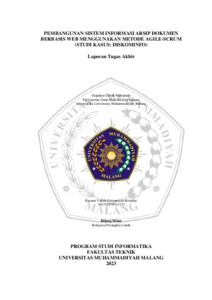## ISOMORFISMA RUANG VEKTOR ATAS SUATU LAPANGAN

AMRY, ULIL (2010) ISOMORFISMA RUANG VEKTOR ATAS SUATU LAPANGAN. Other thesis, University of Muhammadiyah Malang.Preview
Text
ISOMORFISMA_RUANG_VEKTOR__ATAS_SUATU_LAPANGAN.pdf - Published Version

## Abstract

Vector space defined as the set with operations that meet certain axiom. Suppose given a set and a field . Element of called vectors and elements of is called a scalar while the "" and "" respectively denote the addition operation of two vectors and multiplication operations between a vector and scalar. This thesis discusses isomorphism of vector spaces over a field. If given {[ ]| } and {[ ]| } for and is the set matrix with rows and columns , to find out isomorphic to . To determine a vector space isomorphism of the field is done by showing that the mapping is a mapping. Having proved a mapping , the next show that is bijection mapping and homomorphism. The discussion results can be concluded that the vector space over field homomorphism is a mapping which preserves the vector operations in space. While the vector space isomorphism is a special form of vector space homomorphism, ie a mapping which also preserves the vector operations in space and bijection mapping. Vector space which is the set of all matrices of size isomorphic with the vector space of which is also the set of all matrices of size matrix with elements of the field by the mapping for the matrix elements of the vector space while the and are respectively and matrices over . So that for every matrix in a vector space can be determined by a single matrix in the vector space of and vice versa so that the system are twins who can differ only by name and not by nature.

Item Type: Thesis (Other) L Education > L Education (General) Faculty of Teacher Training and Education > Department of Mathematics Education (84202) Anwar Jasin 16 Mar 2012 13:59 16 Mar 2012 13:59 http://eprints.umm.ac.id/id/eprint/516View Item